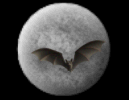God & Science Forum Message Forums: Atm · Astrophotography · Blackholes · Blackholes2 · CCD · Celestron · Domes · Education Eyepieces · Meade · Misc. · God and Science · SETI · Software · UFO · XEphemBe the first pioneers to continue the Astronomy Discussions at our new Astronomy meeting place...The Space and Astronomy Agora More On T Forum List | Follow Ups | Post Message | Back to Thread Topics | In Response ToPosted by Harvey on July 17, 2003 18:37:07 UTC

Michael,

In any case, the term "true" has very specific meaning and definition in math. The definition is given by the symbol '='. The meaning of 'true' in math is the same as the meaning of math itself. Truth, and the equal sign, are meta-concepts in math, as they refer to math itself.

In metalogic and metamathematics, equivalence (or identity) is cited to be a primitive by well-known types (e.g., Alfred Tarski, W.V.O. Quine, etc). If you need references I can provide them. The term 'true' is encased in truth functionals which determine the conditions by which something can be 'T' or 'F'.

Notice how the '=' sign embodies the notion of truth: to say "it is true that 2 + 2 = 4" is exactly equivalent to saying "2 + 2 = 4". "It is true that..." is redundant, as the concept of truth is already expressed by the '=' sign. That is why it makes no sense to question whether "it is true that 2 + 2 = 4", as it is exactly equivalent to asking "given that X is a true assertion, is it true that assertion X is true?".

In fuzzy math (an example of a multi-valued truth value mathematics), the '=' can be replaced by approximate memberships, however the term 'true' remains intact even with fuzzy equivalence, such as:

A~B, I believe, is equivalent as A=B in fuzzy mathematics, but A~B is not the same as A=B in classical math.

I think you are confusing the mathematical notion of truth, as expressed by the '=' sign, with the philosophical notion of truth, which concerns statements about reality. People tend to get confused by that; I suppose it's hard to understand that mathematics is not concerned with reality at all. People learn at a very young age that "you're not supposed to add apples and oranges", when in fact you're not even supposed to add apples and apples. The only thing you can add is numbers. By the time one is old enough to assimilate that, the notion that numbers refer to "things" is too deeply ingrained in one's consciousness.

I don't think so. 'True' in classical mathematics is just a bucket called T, truth, true (whatever you want to call it). There are 2 buckets that results get tossed into. Mathematicians don't try and answer what each bucket is exactly, they just try and determine what conditions are needed in order to toss a result into one of the two buckets. If the conditions aren't specific enough, they go in a bucket called 'undetermined'. Philosophy of truth asks what do the buckets mean in terms of reality. Different issue altogether.